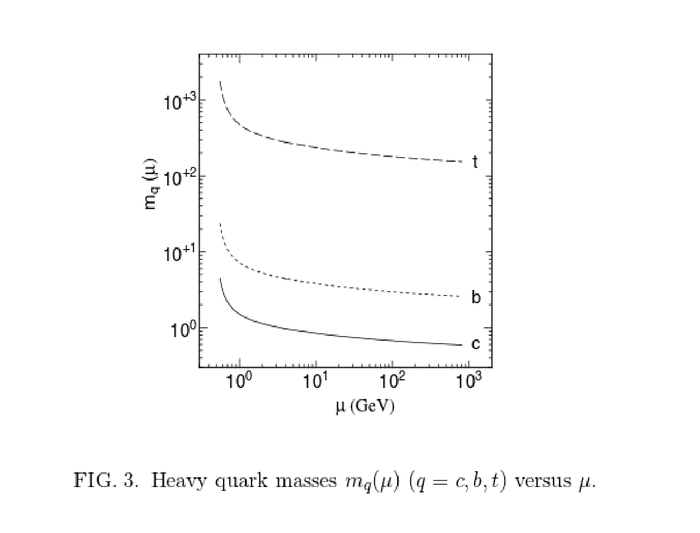# Top quark mass mt at energy scales μ<mt?

Does it make sense to talk about the top mass at energies below mt, although in all processes the corresponding energy scale is above mt because of the rest mass energy of the top quark?

Using an effective field theory approach, the top quark decouples at energies below the top quark mass and therefore has no influence on the mass running of the other fermions. Nevertheless, it is possible to compute how the top quark mass mt changes at energies below mt, for example, because of the energy dependence of the gauge coupling constants. For example, in Updated Estimate of Running Quark Masses the authors computeImagine, for example, the top would be stable for some reason and we could determine its mass somehow using a "scale" at low energies. Would we get the top mass at "room temperature", i.e. a few milli-GeV or only always its mass at around 200 GeV, because of the rest mass energy of the top quark?

Staff Emeritus
Does it make sense to talk about the top mass at energies below mt

How would you ever measure this?

Unfortunately, I have no good idea. Maybe, assuming it's metastable, i.e. with a long but not infinity lifetime, we could bring it to "rest" (cool it to a given temperature) and then sum the energy of the decay products?

mfb
Mentor
The decay process would still happen at the top mass. Unless your top is completely off-shell, but then the result is not the top mass anyway.

Thanks for your answer. My question is motivated by the result that, assuming a fixed standard model vev, one has in SU(5) GUTs at high energies m_bottom=m_tau. However through the RGE running of the Yukawas one gets at low energies m_bottom=3 m_tau. The top mass in the article I cite above is computed at 10^15 GeV to be around 84 GeV and at 2 GeV to be 360 GeV. Assuming the mass of another particle is around 200 GeV at all energy scales, i.e. the corresponding Yukawa coupling runs much slower. Would the top be able to decay into this new particle at low energies where m_new<m_top, but not at high energies where m_new>m_top?

Actually, I think the running of the top mass at scales well below mt has actually been indirectly measured by several processes.

Essentially, all rare B decays (fcnc for example) are mediated by top penguins and boxes. In the predictions of these branching ratios, the top is ran from the top quark pole mass down to mU=mb and used as an input. In some cases, the uncertainty in the top mass as mt, leads to large uncertainties for the process in question. For example, the calculation of B_s-> mU mU at NNLO.

If the top mass were very different to that expected in the SM, it would have also been seen at lep through Z->bb observables too!

ohwilleke
Gold Member
I thought that the SM forbade fcnc interactions

mfb
Mentor
Only at tree level, they appear in higher orders.

Yes, sorry if my post wasn't clear.

In summary, the top quark plays an effect on many observables at scales well below mt. The reason being that these processes are only loop mediated, or the loop mediated contribution is large.

In many cases (like rare Bhadron decays or Z->bb B0 mixing etc.) the effects from the top are very significant. In fact, maybe first estimates of the top mass (before direct observation) came from theory predictions of these observable s compared to data, where they extract the top mass.

To do this, generally the msbar (running top mass is used). In the case of B decays, an appropriate scale choice of mu is around the b quark mass.

Hope this helps